# Talk about quantifying investment using Python

2022-01-30 12:07:54 ReganYue

### 0x00 Preface

Quantitative trading uses computer technology （ This article mainly refers to the use of Python） Help investors analyze a large amount of data to formulate investment strategies , This belongs to the cross field of Finance and computer .

This article is used to guide the use of Python Beginners who carry out quantitative transactions get started , Limited to my limited level , Let's take it easy ~

### 0x01 Extract the data

#### precondition

To extract stock data , We need to use Quandl Of API, This platform has a lot of economic and financial data .

First we need to install Python 3 and virtualenv, And pass

``````virtualenv --python=/usr/bin/python3 <name of env>
Copy code ``````

Creating a virtual environment .

And then use

``````source <env_name>/bin/activate
Copy code ``````

Activate the virtual environment .

Reuse pip install jupyter-notebook：

``````pip install jupyter-notebook
Copy code ``````

And then install it `pandas`,`quandl` and `numpy` package .

Last run jupyter-notebook.

#### Extract the data

Let's import the required package first .

``````import pandas as pd
import quandl as q
Copy code ``````

here pandas It can help us with data operation and drawing .

Then we call Quandl API.

``````q.ApiConfig.api_key = "<API key>”
​
msft_data = q.get("EOD/MSFT", start_date="2010-01-01", end_date="2019-01-01")
​
Copy code ``````

In this code , We first set the required api_key. You need to go to the official website to get .

And then call get Method to get Microsoft from 2010 year 1 month 1 The day is coming 2019 year 1 month 1 The stock price on the day .

Then there is the front view of the acquired dataset 5 That's ok .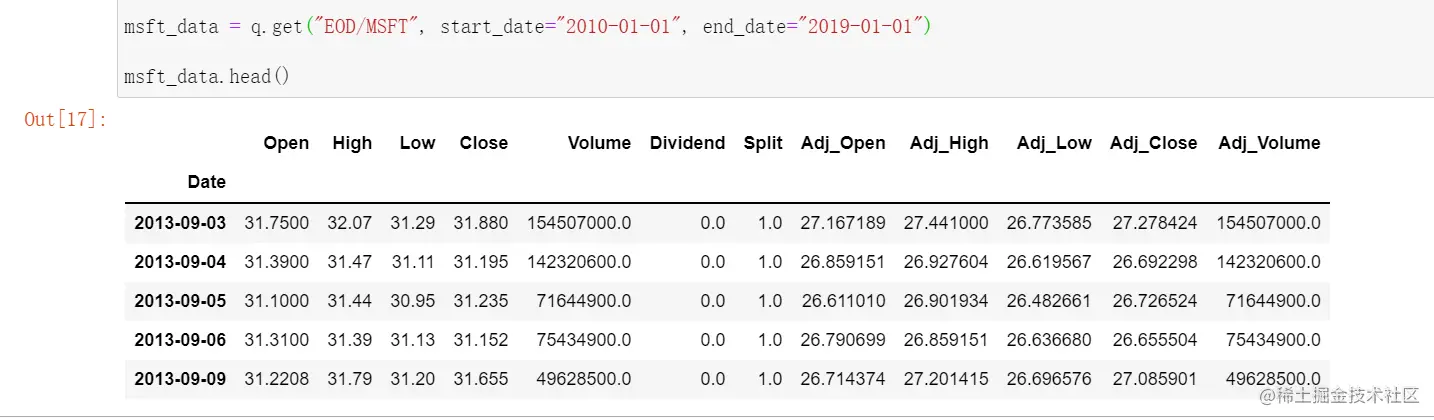### 0x02 Analyze the data

Let's first understand the data composition of the dataset , This can be done through info Method view .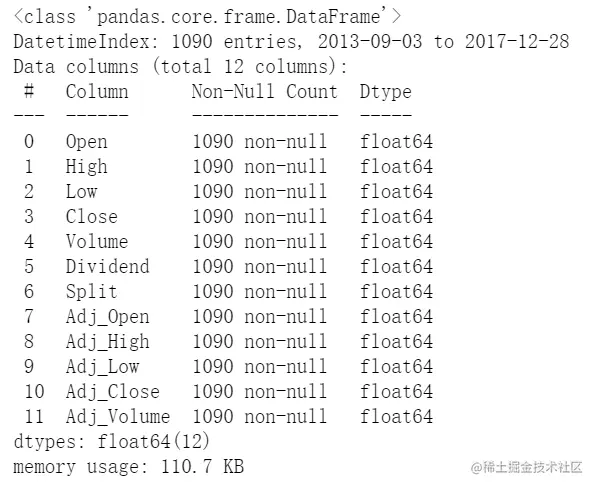As shown in the figure above , We can see that these data contain stock price information .

Such as the opening price and closing price of the stock 、 Adjusted opening and closing prices , This adjustment is to distribute dividends 、 Stock splits and other actions are taken into account , It also includes the trading volume and the highest and lowest prices of the day .

We can also go through describe Method to analyze the maximum value of the data set 、 minimum value 、 Average and other data .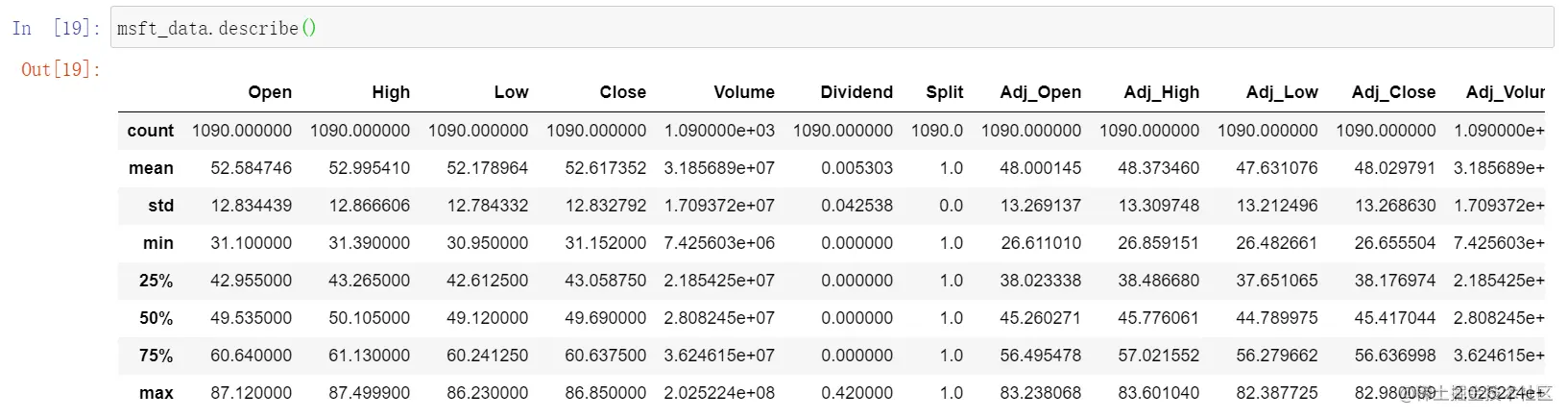We can also continue to process these data .

For example, take another sample . Adjust the time interval to monthly 、 Quarterly or annually .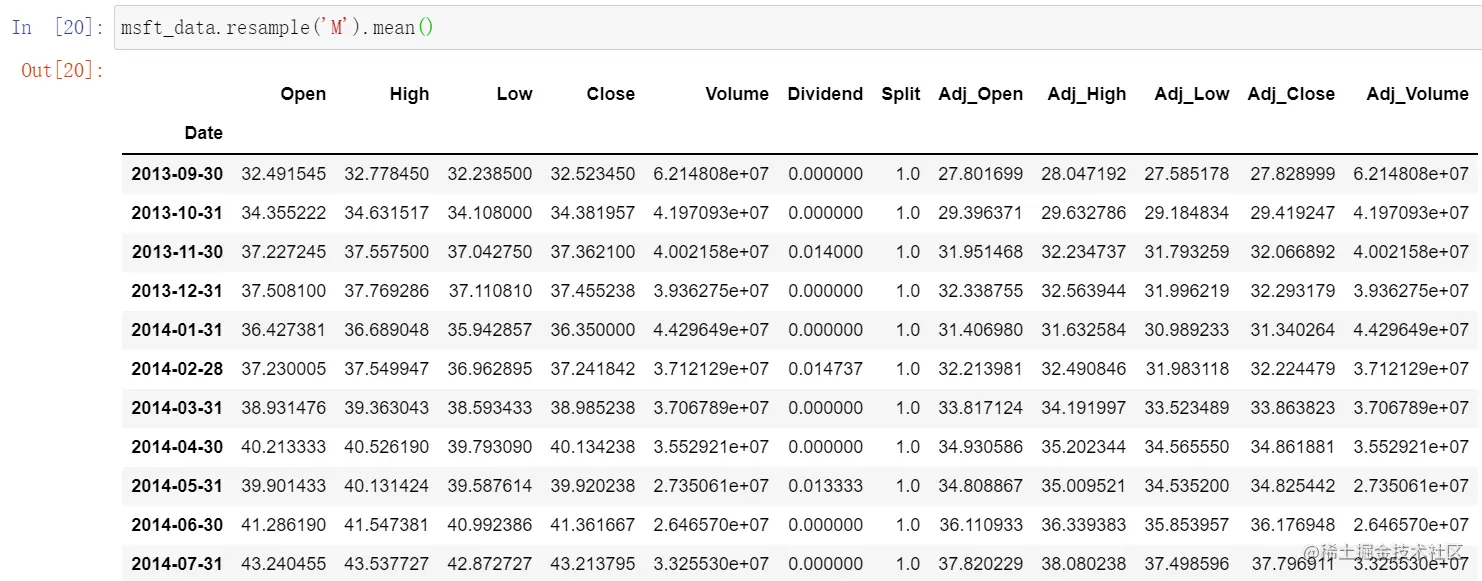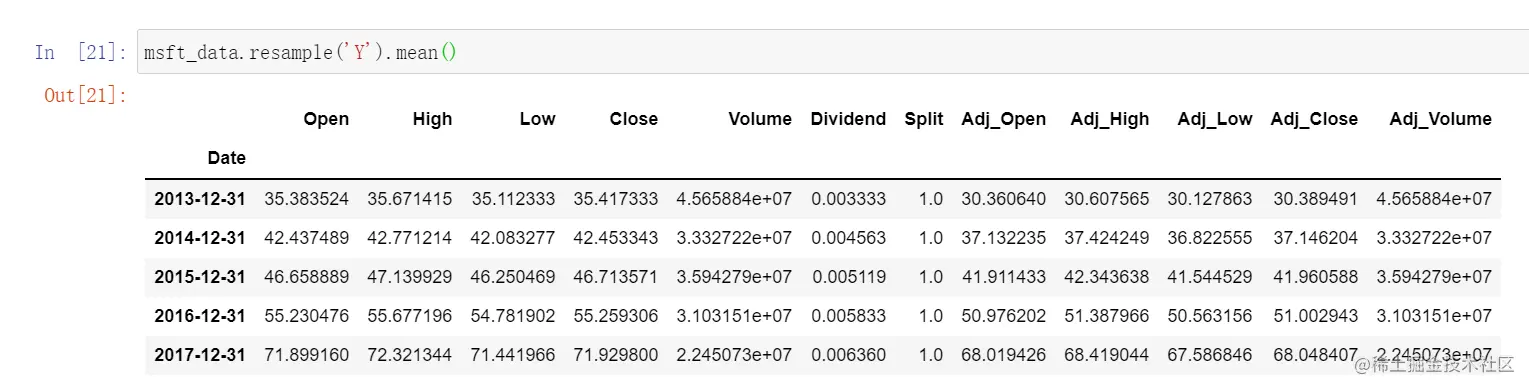### 0x04 Calculate financial returns

Let's next calculate the financial return .

We're going to use pct_change() Method .

``````import numpy as np
​
​
daily_return = daily_close.pct_change()
​
daily_return.fillna(0, inplace=True)
​
print(daily_return)
Copy code ``````

We use the adjusted closing price to calculate the return from the start time to each time . Multiply this value 100 You get a percentage return .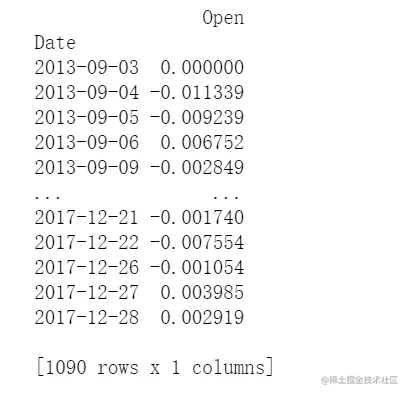We can also calculate the rate of return with a time interval of months ：

``````mdata = msft_data.resample('M').apply(lambda x: x[-1])
monthly_return = mdata.pct_change()
monthly_return.fillna(0, inplace=True)
print(monthly_return)
Copy code ``````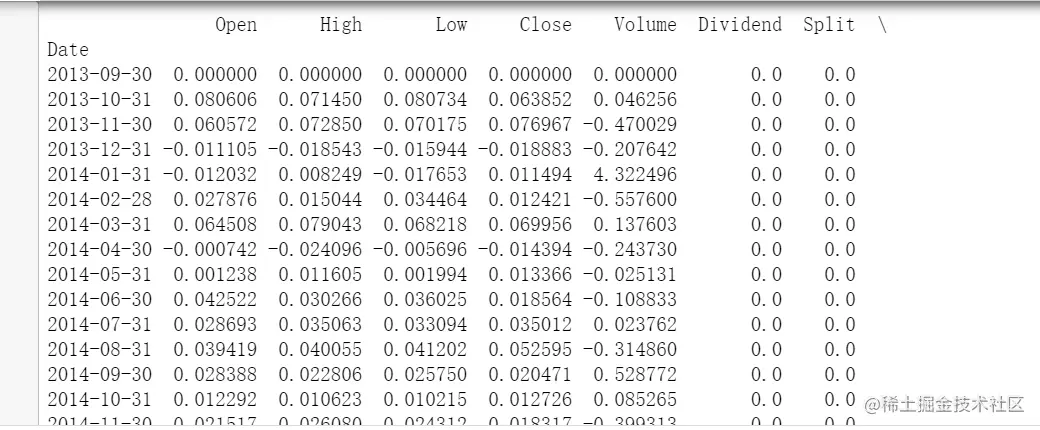Some codes refer to ：www.codementor.io/blog/quanti…